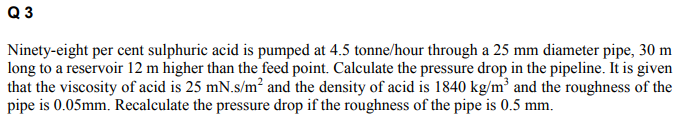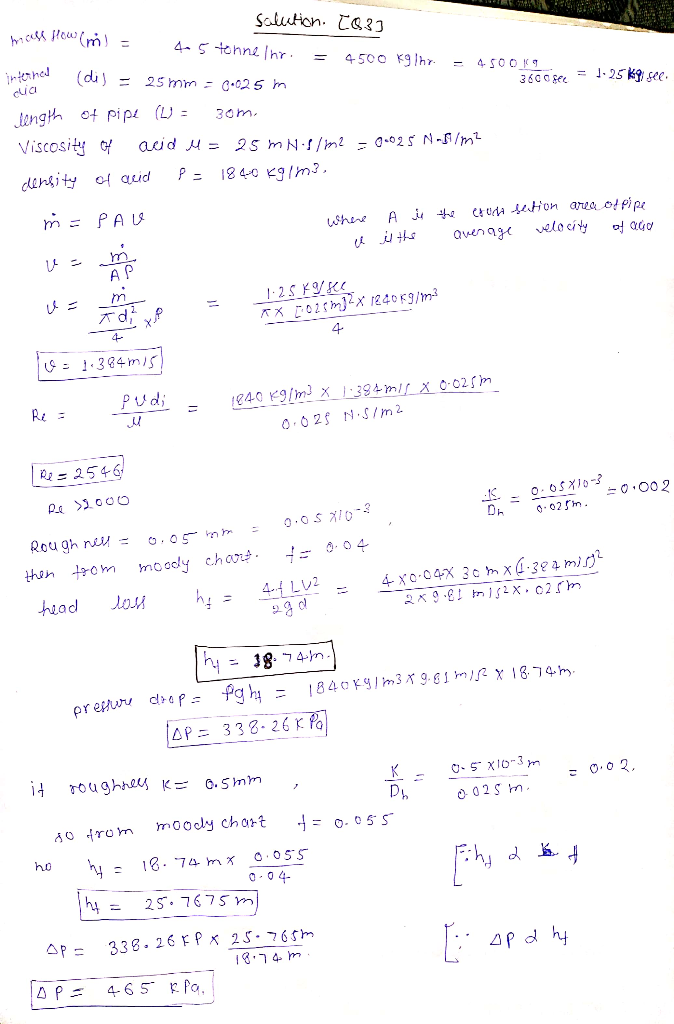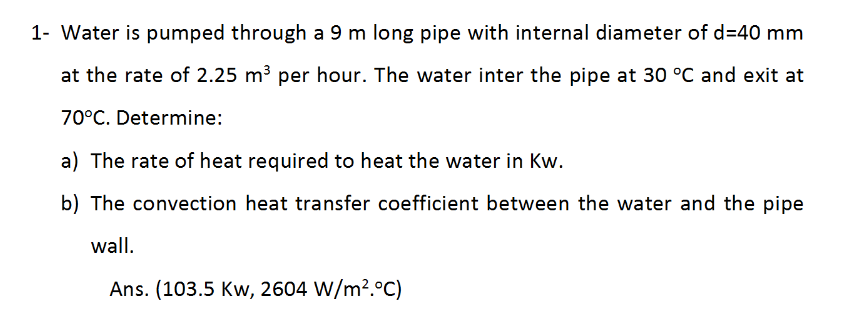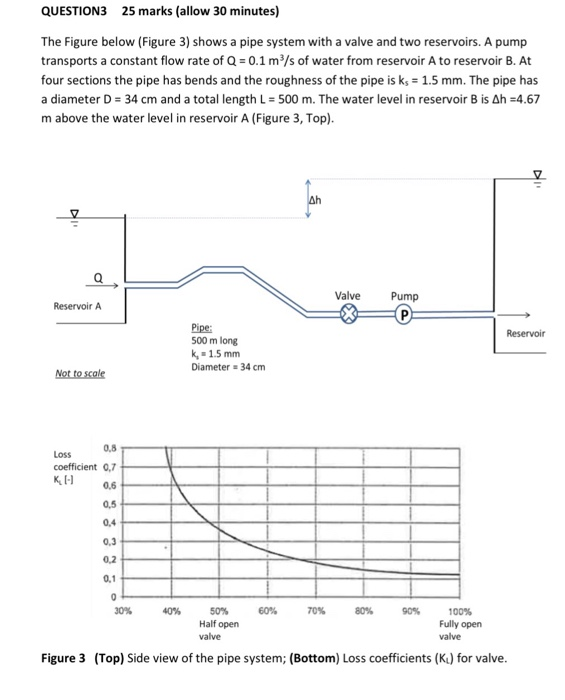# Q 3 Ninety-eight per cent sulphuric acid is pumped at 4.5 tonne/hour through a 25 mm diameter pip...Q 3 Ninety-eight per cent sulphuric acid is pumped at 4.5 tonne/hour through a 25 mm diameter pipe, 30 m long to a reservoir 12 m higher than the feed point. Calculate the pressure drop in the pipeline. It is given that the viscosity of acid is 25 mN.s/m2 and the density of acid is 1840 kg/m3 and the roughness of the pipe is 0.05mm. Recalculate the pressure drop if the roughness of the pipe is 0.5 mm.##### Add Answer of: Q 3 Ninety-eight per cent sulphuric acid is pumped at 4.5 tonne/hour through a 25 mm diameter pip...
Similar Homework Help Questions
• ### 1- Water is pumped through a 9 m long pipe with internal diameter of d-40 mm at the rate of 2.25 m3 per hour. The water inter the pipe at 30 °C and exit at 70°C. Determine: b) The convection heat tra...1- Water is pumped through a 9 m long pipe with internal diameter of d-40 mm at the rate of 2.25 m3 per hour. The water inter the pipe at 30 °C and exit at 70°C. Determine: b) The convection heat transfer coefficient between the water and the pipe wall Ans. (103.5 Kw, 2604 W/m2.°C) 1- Water is pumped through a 9 m long pipe with internal diameter of d-40 mm at the rate of 2.25 m3 per hour. The...

• ### QUESTION3 25 marks (allow 30 minutes) The Figure below (Figure 3) shows a pipe system with a valve and two reservoirs. A pump transports a constant flow rate of Q 0.1 m3/s of water from reservoi...QUESTION3 25 marks (allow 30 minutes) The Figure below (Figure 3) shows a pipe system with a valve and two reservoirs. A pump transports a constant flow rate of Q 0.1 m3/s of water from reservoir A to reservoir B. At four sections the pipe has bends and the roughness of the pipe is ks 1.5 mm. The pipe has a diameter D 34 cm and a total length L = 500 m. The water level in reservoir B is...

Free Homework App